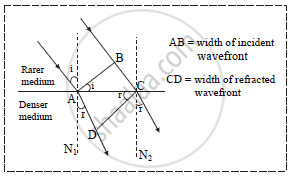Share

# The Width of Plane Incident Wavefront is Found to Be Doubled on Refraction in Denser Medium. If It Makes an Angle of 65° Medium. with the Normal, Calculate the Refractive Index for the Denser Medium. - Physics

#### Question

The width of plane incident wavefront is found to be doubled on refraction in denser medium. If it makes an angle of 65° medium. with the normal, calculate the refractive index for the denser medium.

#### SolutionGiven: i = 65°, CD = 2AB
To find: Refractive index (μ)

formulae:

cosi/cosr="AB"/"CD" ..........(1)

mu=sini/sinr ...................(2)

From formula 1

cos65^@/cosr="AB"/"2AB"

0.4226/cosr=1/2

cosr=32^@16'

from formula 2

mu=sin65^@/sin(32^@16')

mu=1.697

The refractive index for the denser medium is 1.697.

Is there an error in this question or solution?

#### APPEARS IN

2016-2017 (March) (with solutions)
Question 8.1 | 7.00 marks
Solution The Width of Plane Incident Wavefront is Found to Be Doubled on Refraction in Denser Medium. If It Makes an Angle of 65° Medium. with the Normal, Calculate the Refractive Index for the Denser Medium. Concept: Measurement of Wavelength by Biprism Experiment.
S